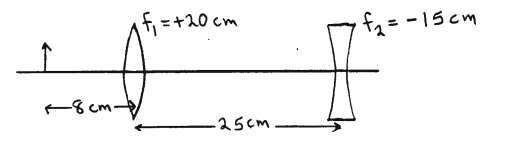# Problem: A converging with focal length f1 = +20.0 cm is 25.0 cm to the left of a diverging lens that has focal length f2 = -15.0 cm. An object that is 2.0 mm tall is placed 8.00 cm to the left of the converging lens. How far is the final image from the diverging lens?

###### FREE Expert Solution
80% (294 ratings)
###### Problem Details

A converging with focal length f= +20.0 cm is 25.0 cm to the left of a diverging lens that has focal length f= -15.0 cm. An object that is 2.0 mm tall is placed 8.00 cm to the left of the converging lens. How far is the final image from the diverging lens?